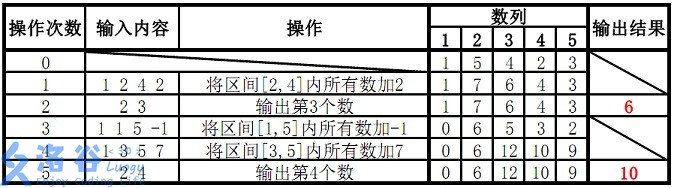【luogu3368】【模板】树状数组 2

1.将某区间每一个数数加上x

2.求出某一个数的和

```5 5
1 5 4 2 3
1 2 4 2
2 3
1 1 5 -1
1 3 5 7
2 4```

```6
10```

说明1A杠杠的

 1 2 3 4 5 6 7 8 9 10 11 12 13 14 15 16 17 18 19 20 21 22 23 24 25 26 27 28 29 30 31 32 33 34 35 36 37 38 39 40 41 42 43 44 45 46 47 48 49 50 51 52 `#include` `#include` `#include` `#define MAXN 1000000` `using namespace std;` `int n, m;` `int a[MAXN];` `int e[MAXN];`   `int lowbit(int x){` `    ``return` `x & -x;` `}`   `void add(int i, int x){` `    ``while``(i <= n){` `        ``a[i] += x;` `        ``i += lowbit(i);` `    ``}` `    ``return``;` `}`   `int sum(int k){` `    ``int res = 0;` `    ``while``(k > 0){` `        ``res += a[k];` `        ``k -= lowbit(k);` `    ``}` `    ``return` `res;` `}`   `int main(){` `    ``scanf(``"%d%d"``, &n, &m);` `    ``memset(a, 0, sizeof(a));` `    ``e = 0;` `    ``for` `(int i = 1; i <= n; i++){` `        ``scanf(``"%d"``, &e[i]);` `        ``add(i, e[i] - e[i - 1]);` `    ``}` `    ``int x, y, k;` `    ``while``(m--){` `        ``scanf(``"%d"``, &x);` `        ``if` `(x == 1){` `            ``scanf(``"%d%d%d"``, &x, &y, &k);` `            ``add(x, k);` `            ``add(y + 1, -k);` `        ``}``else` `if` `(x == 2){` `            ``scanf(``"%d"``, &k);` `            ``printf(``"%d\n"``, sum(k));` `        ``}` `    ``}` `    ``return` `0;` `}`